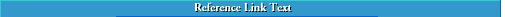## Enumeration

Unless you are following a style guide that specifies otherwise, observe the following conventions.

Use enumeration in reports and other documents to identify sequences of chapters, sections, page numbers, figures and tables, equations, footnotes, and appendixes. Lengthy reports may contain and enumerate all these items. Any technical or scientific document of more than one page, however, will at least enumerate its pages, as well as any other of these elements that are present.

#### Chapter-Section Enumeration

Of the two general enumeration systems widely used, the numerical system is clearer than the alphanumerical system. In the numerical system, the reader can always locate his or her place in the document from the single decimal number.

```NUMERICAL SYSTEM    ALPHANUMERICAL SYSTEM
1                   I.
1.1                      A.
1.2                      B.
1.2.1                         1.
1.2.2                         2.
2                   II.
2.1                      A.
2.1.1                         1.
2.1.2                         2.
2.2                      B.
```

#### Pagination

Number the front matter in italic lowercase roman numerals (i, ii, iii, iv, and so on).

Normally, number the pages in the body of the document with arabic numerals, starting with page 1. Number sequentially through page n at the end of the text, including all back matter. Some long reports and theses, however, employ a two-part numbering system that contains a numerical chapter prefix followed by a hyphen and then the page number of the chapter. The first page of Chapter 6, for example, would be numbered 6-1.

#### Tables and Figures

Number tables and figures sequentially as Table 1, Table 2, Table 3, and so on. In long documents, however, table and figure numbers are often prefixed with a chapter number: Table 5-1 or Table 5.1, for example, refers to the first table of Chapter 5.

#### Equations

Number all equations discussed in the text sequentially, as Eq. 1, Eq. 2, Eq. 3, and so on. You do not have to number every equation that appears in the document because many equations are part of a series of intermediate steps that are not specifically discussed.

#### Footnotes, Endnotes, and Reference Numbers

See the discussion in Citing Sources and Listing References.## Enumeration and Lists ##